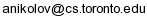## Course Information

 Instructor Sasho Nikolov E-mail:Additional Reading Matousek's Geometric Discrepancy Theory beautifully covers the classical theory. Also check Chazelle's The Discrepancy Method, available freely from his website.

## Course Description

Discrepancy theory is an area of mathematics that studies how well discrete objects can approximate continuous ones. It has deep connections to number theory, harmonic analysis, combinatorics, computer science. Some typical questions are: What is the most uniform set of n points in the unit square?; Given a set of n points in the plane, can we color them red and blue so that every halfplane contains roughly equal numbers of red and blue points?; Can we round a solution to a linear program to integers so that it stays approximately feasible?

Interestingly these questions can be addressed using a common set of tools. In this course we will present some of them, focusing on:

• combinatorial methods;
• algorithmic proofs, i.e. proofs that also give efficient constructions;
• applications to computer science.

## Lecture notes

The lecture notes below are based on scribe notes by Lalla Moutadid, Robert Robere, and Asseimakis Kattis.

1. [Notes] Basic notions: continuous discrepancy, combinatorial discrepancy, matrix discrepancy, transference theorems.
2. [Notes] Spencer's theorem: Lovett and Meka's proof of the Six Standard Deviations Suffice theorem.
3. [Notes] The Beck-Fiala Setting: The Beck-Fiala theorem, and algorithmic proof of the Banaszczyk bound.
4. [Notes] Approximating Hereditary Discrepancy: efficiently approximating hereditary discrepancy using the γ2 norm.
5. [Notes] An Application to Approximation Algorithms: rounding via discrepancy, and an efficient approximation algorithm for the bin-packing problem.

Please attempt the exercises in the lecture notes above.